SEARCH HOMEMath Central Quandaries & QueriesQuestion from chelsie, a student: how do i find the perimeter of a triangle given the area, base, and height?We havve two responses for you.

Hi Chelsie,

There are many different perimeters possible if all you know is the area, base and height. Let's look at a particular example with the base = b = 2 cm and the height = h = 1 cm. The area is then

1/2 b ×h = 1 cubic centimeter.

This might be a right triangle.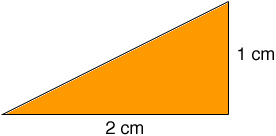Pythagoras' theorem give us the length of the hypotenuse as sqrt(12 + 22) = √5 cm and hence the triangle has perimeter 3 + √5 which is approximately 5.236 cm.

It might be an isosceles triangle.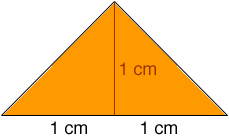This is two right triangles, each with hypotenuse of length sqrt(12 + 12) = √2 cm and hence the triangle has perimeter 2 + 2√2 which is approximately 4.828 cm.

It might be neither a right triangle nor an isosceles triangle.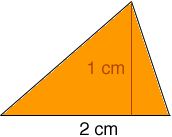The perimeter depends on the exact shape of the triangle.

Penny

The perimeter is still not determined. The base and height determine the area, but ... See the three pictures below.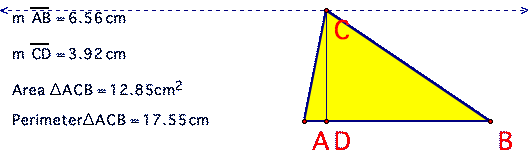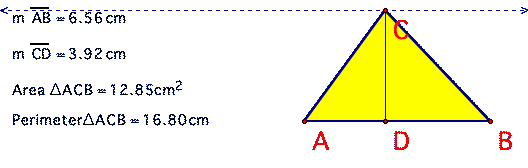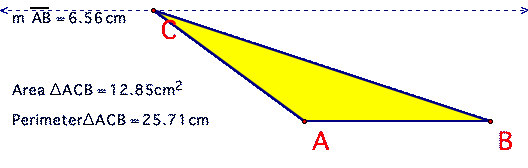You might make this into an investigation, by considering:
- when the perimeter is the smallest?
- how big the perimeter can become for this area, base and height!

Walter WhiteleyMath Central is supported by the University of Regina and The Pacific Institute for the Mathematical Sciences.